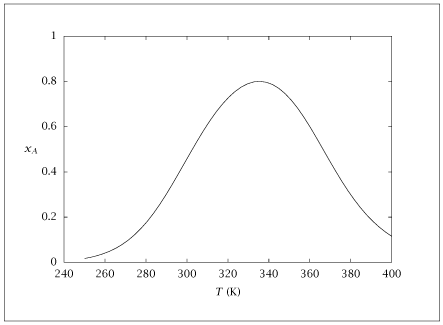## Figure 6.4:

### Conversion of A versus reactor temperature.## Code for Figure 6.4

### main.m

```
1
2
3
4
5
6
7
8
9
10
11
12
13
14
15
16
17
18
19
20
21
22
23
24
25
26
27
28
29
30
31
32
33
34
35
36
37
38
39
40
41
42
43
44
45
46
47
48
49
50
51
52
53
54
55
56
57
58
59% Copyright (C) 2001, James B. Rawlings and John G. Ekerdt
%
% This program is free software; you can redistribute it and/or
% modify it under the terms of the GNU General Public License as
% published by the Free Software Foundation; either version 2, or (at
% your option) any later version.
%
% This program is distributed in the hope that it will be useful, but
% WITHOUT ANY WARRANTY; without even the implied warranty of
% MERCHANTABILITY or FITNESS FOR A PARTICULAR PURPOSE.  See the GNU
% General Public License for more details.
%
% You should have received a copy of the GNU General Public License
% along with this program; see the file COPYING.  If not, write to
% the Free Software Foundation, 59 Temple Place - Suite 330, Boston,
% MA 02111-1307, USA.
%
% Revised 8/22/2018

%
% parameters; units are: kcal, lit, mol, min
%
k_10      = 3e7;
E_1       = 5838;
K_10      = 1.9e-11;
G_1       = -9059;
c_Af      = 4;
Q_f       = 250;
%DeltaH_R = -18;
V_R      = 2000;
conv     = 0.8;

% function retval = st_st_cA(x)
%   T     = x(1);
%   k_1   = k_10*exp(-E_1/T);
%   K_1   = K_10*exp(-G_1/T);
%   c_A   = c_Af*(1-conv);
%   c_R   = c_Af - c_A;
%   retval(1) = Q_f/V_R*(c_Af-c_A) - k_1*(c_A - 1/K_1*c_R);
% end%function
%
% T0 = 300;
% [T, fval, info] = fsolve('st_st_cA', T0);
%

T = linspace(250,400,50)';
k_1 = k_10*exp(-E_1./T);
K_1 = K_10*exp(-G_1./T);

c_A  = (Q_f/V_R + k_1./K_1)*c_Af ./ (Q_f/V_R + k_1.*(1 + 1./K_1));
convvec = (c_Af - c_A)/c_Af;
table = [T c_A convvec];

save -ascii reversible_cstr.dat table;

if (~ strcmp (getenv ('OMIT_PLOTS'), 'true')) % PLOTTING
plot (table(:,1),table(:,3));
% TITLE
end % PLOTTING

```